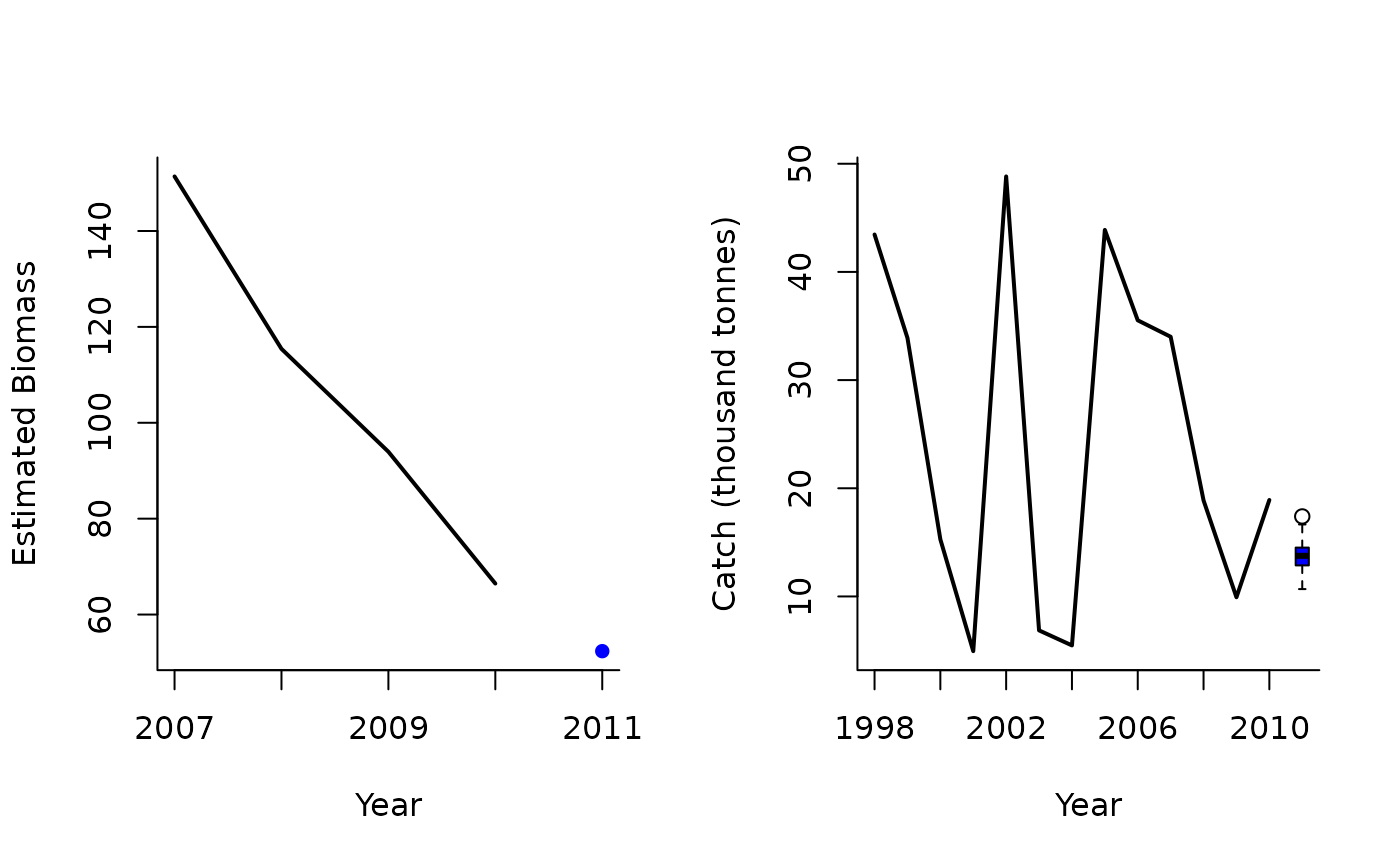A management procedure that makes incremental adjustments to TAC recommendations based on the apparent trend in recent surplus production. Based on the theory of Mark Maunder (IATTC)

SPslope(
x,
Data,
reps = 100,
plot = FALSE,
yrsmth = 4,
alp = c(0.9, 1.1),
bet = c(1.5, 0.9)
)

## Arguments

x

A position in the data object

Data

A data object

reps

The number of stochastic samples of the MP recommendation(s)

plot

Logical. Show the plot?

yrsmth

Years over which to smooth recent estimates of surplus production

alp

Condition for modifying the Data (bounds on change in abundance)

bet

Limits for how much the Data can change among years

## Value

An object of class Rec-class with the TAC slot populated with a numeric vector of length reps

## Details

Note that this isn't exactly what Mark has previously suggested and is stochastic in this implementation.

The TAC is calculated as: $$\textrm{TAC}_y = \left\{\begin{array}{ll} M \bar{C} & \textrm{if } r < \alpha_1 \\ \bar{C} & \textrm{if } \alpha_1 < r < \alpha_2 \\ \textrm{bet}_2 \textrm{SP} & \textrm{if } r > \alpha_2 \\ \end{array}\right.$$ where $$r$$ is the ratio of predicted biomass in next year to biomass in current year $$\bar{C}$$ is the mean catch over the last yrmsth years, $$\alpha_1$$ and $$\alpha_2$$ are specified in alp, $$\textrm{bet}_1$$ and $$\textrm{bet}_2$$ are specified in bet, $$\textrm{SP}$$ is estimated surplus production in most recent year, and: $$M = 1-\textrm{bet}_1 \frac{B_y - \tilde{B}_y}{B_y}$$ where $$B_y$$ is the most recent estimate of biomass and $$\tilde{B}$$ is the predicted biomass in the next year.

## Required Data

See Data-class for information on the Data object

SPslope: Abun, Cat, Ind, Year

## Rendered Equations

See Online Documentation for correctly rendered equations

Other Surplus production MPs: Fadapt(), Rcontrol(), SPMSY(), SPSRA(), SPmod()

T. Carruthers

## Examples

SPslope(1, Data=MSEtool::Atlantic_mackerel, plot=TRUE)#> TAC (median)
#>     13.90378